# Test: Distance And Speed- 3

## 10 Questions MCQ Test Practice Questions for GMAT | Test: Distance And Speed- 3

Description
Attempt Test: Distance And Speed- 3 | 10 questions in 20 minutes | Mock test for GMAT preparation | Free important questions MCQ to study Practice Questions for GMAT for GMAT Exam | Download free PDF with solutions
QUESTION: 1

### Bob bikes to school every day at a steady rate of x miles per hour. On a particular day, Bob had a flat tire exactly halfway to school. He immediately started walking to school at a steady pace of y miles per hour. He arrived at school exactly t hours after leaving his home. How many miles is it from the school to Bob's home?

Solution:

Let b be the number of hours Bob spends biking. Then (t – b) is the number of hours he spends walking. Let d be the distance in miles from his home to school. Since he had the flat tire halfway to school, he biked d/2 miles and he walked d/2 miles. Now we can set up the equations using the formula rate x time = distance. Remember that we want to solve for d, the total distance from Bob's home to school.

1) xb = d/2
2) y(t – b) = d/2

Solving equation 1) for b gives us:

3) b = d/2x                 Substituting this value of b into equation 2 gives:

4) y(t – d/2x) = d/2    Multiply both sides by 2x:

5) 2xy(t – d/2x) = dx  Distribute the 2xy

6) 2xyt – dy = dx       Add dy to both sides to collect the d's on one side.

7) 2xyt = dx + dy       Factor out the d

8) 2xyt = d(x + y)      Divide both sides by (x + y) to solve for d

9) 2xyt / (x + y) = d

QUESTION: 2

### Lexy walks 5 miles from point A to point B in one hour, then bicycles back to point A along the same route at 15 miles per hour. Ben makes the same round trip, but does so at half of Lexy’s average speed. How many minutes does Ben spend on his round trip?

Solution:

We begin by figuring out Lexy’s average speed. On her way from A to B, she travels 5 miles in one hour, so her speed is 5 miles per hour. On her way back from B to A, she travels the same 5 miles at 15 miles per hour. Her average speed for the round trip is NOT simply the average of these two speeds. Rather, her average speed must be computed using the formula RT = D, where R is rate, T is time and D is distance. Her average speed for the whole trip is the total distance of her trip divided by the total time of her trip.

We already know that she spends 1 hour going from A to B. When she returns from B to A, Lexy travels 5 miles at a rate of 15 miles per hour, so our formula tells us that 15T = 5, or T = 1/3. In other words, it only takes Lexy 1/3 of an hour, or 20 minutes, to return from B to A. Her total distance traveled for the round trip is 5+5=10 miles and her total time is 1+1/3=4/3 of an hour, or 80 minutes.

We have to give our final answer in minutes, so it makes sense to find Lexy's average rate in miles per minute, rather than miles per hour. 10 miles / 80 minutes = 1/8 miles per minute. This is Lexy's average rate.

We are told that Ben's rate is half of Lexy's, so he must be traveling at 1/16 miles per minute. He also travels a total of 10 miles, so (1/16)T = 10, or T = 160. Ben's round trip takes 160 minutes.

Alternatively, we could use a shortcut for the last part of this problem. We know that Ben's rate is half of Lexy's average rate. This means that, for the entire trip, Ben will take twice as long as Lexy to travel the same distance. Once we determine that Lexy will take 80 minutes to complete the round trip, we can double the figure to get Ben's time. 80 × 2 = 160.

QUESTION: 3

### Triathlete Dan runs along a 2-mile stretch of river and then swims back along the same route. If Dan runs at a rate of 10 miles per hour and swims at a rate of 6 miles per hour, what is his average rate for the entire trip in miles per minute?

Solution:

There is an important key to answering this question correctly: this is not a simple average problem but a weighted average problem.  A weighted average is one in which the different parts to be averaged are not equally balanced.  One is "worth more" than the other and skews the "simple" average in one direction.  In addition, we must note a unit change in this problem: we are given rates in miles per hour but asked to solve for rates in miles per minute.
Average rate uses the same D = RT formula we use for rate problems but we have to figure out the different lengths of time it takes Dan to run and swim along the total 4-mile route. Then we have to take the 4 miles and divide by that total time. First, Dan runs 2 miles at the rate of 10 miles per hour.  10 miles per hour is equivalent to 1 mile every 6 minutes, so Dan takes 12 minutes to run the 2 miles.  Next, Dan swims 2 miles at the rate of 6 miles per hour.  6 miles per hour is equivalent to 1 mile every 10 minutes, so Dan takes 20 minutes to swim the two miles.
Dan's total time is 12 + 20 = 32 minutes.  Dan's total distance is 4 miles.  Distance / time = 4 miles / 32 minutes = 1/8 miles per minute.
Note that if you do not weight the averages but merely take a simple average, you will get 2/15, which corresponds to incorrect answer choice B.  6 mph and 10 mph average to 8mph.  (8mph)(1h/60min) = 8/60 miles/minute or 2/15 miles per minute.

QUESTION: 4

Tom and Linda stand at point A. Linda begins to walk in a straight line away from Tom at a constant rate of 2 miles per hour. One hour later, Tom begins to jog in a straight line in the exact opposite direction at a constant rate of 6 miles per hour. If both Tom and Linda travel indefinitely, what is the positive difference, in minutes, between the amount of time it takes Tom to cover half of the distance that Linda has covered and the amount of time it takes Tom to cover twice the distance that Linda has covered?

Solution:

The formula to calculate distance is Distance = (Rate)(Time). So at any given moment Tom's distance (let's call it DT) can be expressed as DT  = 6T. So, at any given moment, Linda's distance (let's call it DL) can be expressed as DL = 2(T + 1) (remember, Linda's time is one hour more than Tom's). The question asks us to find the positive difference between the amount of time it takes Tom to cover half of Linda's distance and the time it takes him to cover twice her distance.  Let's find each time separately first.

When Tom has covered half of Linda's distance, the following equation will hold: 6T = (2(T + 1))/2. We can solve for T:
6T = (2(T + 1))/2
6T = (2T + 2)/2
6T = T +1
5T = 1
T = 1/5

So it will take Tom 1/5 hours, or 12 minutes, to cover half of Linda's distance. When Tom has covered twice Linda's distance, the following equation will hold: 6T = 2(2(T + 1). We can solve for T:

6T = 2(2(T + 1)
6T = 2(2T + 2)
6T = 4T + 4
2T = 4
T = 2

So it will take Tom 2 hours, or 120 minutes, to cover twice Linda's distance.
We need to find the positive difference between these times: 120 – 12 = 108.

QUESTION: 5

It takes the high-speed train x hours to travel the z miles from Town A to Town B at a constant rate, while it takes the regular train y hours to travel the same distance at a constant rate. If the high-speed train leaves Town A for Town B at the same time that the regular train leaves Town B for Town A, how many more miles will the high-speed train have traveled than the regular train when the two trains pass each other?

Solution:

A question with variables in the answer choices (VIC) can be solved by picking values for the variables.

Let's pick the following values for x, y and z: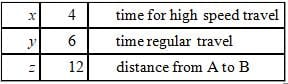When picking values for a VIC question, it is best to pick numbers that are easy to work with (i.e., 12 is divisible by 4 and 6 here), but that don't have any extraneous relationships between them. For example x = 4, y = 3, z = 12 would be a less favorable set of numbers because xy would equal z in that case and there is no need for the product of the two times to equal the distance. Picking variables with extraneous relationships can lead to false positives when checking the answer choices.

Now let's solve the question according to the values we selected.
If the high-speed train travels the 12 miles from A to B in 4 hours, it is traveling at 3 mph.
If the regular train travels the 12 miles from A to B in 6 hours, it is traveling at 2 mph.

To evaluate how far each train travels when they move toward each other starting at opposite ends, let's set up an RTD chart.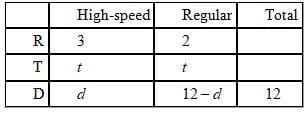We can set-up two equations with two unknowns and solve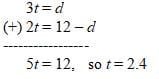In the 2.4 hours it takes for the two trains to meet,
the high speed train will have traveled 3(2.4) = 7.2 miles,
and the regular train will have traveled 2(2.4) = 4.8 miles.
Therefore the high speed train will have traveled 7.2 – 4.8 = 2.4 miles farther than the regular train.
2.4 is our target number.

Let's see which of the five answer choices give us 2.4 when we plug in our values for x, y and z: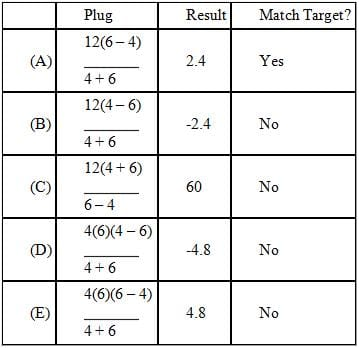Only A matches the target.

This question can also be solved algebraically.
Since the trains traveled the z miles in x and y hours, their speeds can be represented as z/x and z/y respectively.

We can again use an RTD chart to evaluate how far each train travels when they move toward each other starting at opposite ends. Instead of using another variable d here, let's express the two distances in terms of their respective rates and times.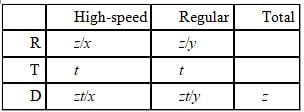Since the two distances sum to the total when the two trains meet, we can set up the following equation:

zt/x + zt/y = z  -      divide both sides of the equation by z
t/x + t/y = 1      -   multiply both sides of the equation by xy
ty + tx = xy    -     factor out a t on the left side
t(x + y) = xy    -   divide both sides by x + y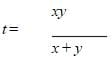To find how much further the high-speed train went in this time:
(ratehigh × time) – (ratereg × time)
(ratehigh ratereg) × time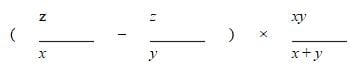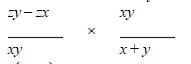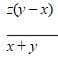QUESTION: 6

The ‘moving walkway’ is a 300-foot long conveyor belt that moves continuously at 3 feet per second. When Bill steps on the walkway, a group of people that are also on the walkway stands 120 feet in front of him. He walks toward the group at a combined rate (including both walkway and foot speed) of 6 feet per second, reaches the group of people, and then remains stationary until the walkway ends. What is Bill’s average rate of movement for his trip along the moving walkway?

Solution:

To determine Bill’s average rate of movement, first recall that Rate × Time = Distance. We are given that the moving walkway is 300 feet long, so we need only determine the time elapsed during Bill’s journey to determine his average rate.

There are two ways to find the time of Bill’s journey. First, we can break down Bill’s journey into two legs: walking and standing. While walking, Bill moves at 6 feet per second. Because the walkway moves at 3 feet per second, Bill’s foot speed along the walkway is 6 – 3 = 3 feet per second. Therefore, he covers the 120 feet between himself and the bottleneck in (120 feet)/(3 feet per second) = 40 seconds.

Now, how far along is Bill when he stops walking? While that 40 seconds elapsed, the crowd would have moved (40 seconds)(3 feet per second) = 120 feet. Because the crowd already had a 120 foot head start, Bill catches up to them at 120 + 120 = 240 feet. The final 60 feet are covered at the rate of the moving walkway, 3 feet per second, and therefore require (60 feet)/(3 feet per second) = 20 seconds. The total journey requires 40 + 20 = 60 seconds, and Bill’s rate of movement is (300 feet)/(60 seconds) = 5 feet per second.

This problem may also be solved with a shortcut. Consider that Bill’s journey will end when the crowd reaches the end of the walkway (as long as he catches up with the crowd before the walkway ends). When he steps on the walkway, the crowd is 180 feet from the end. The walkway travels this distance in (180 feet)/(3 feet per second) = 60 seconds, and Bill’s average rate of movement is (300 feet)/(60 seconds) = 5 feet per second.

QUESTION: 7

John and Jacob set out together on bicycle traveling at 15 and 12 miles per hour, respectively. After 40
minutes, John stops to fix a flat tire. If it takes John one hour to fix the flat tire and Jacob continues to ride during this time, how many hours will it take John to catch up to Jacob assuming he resumes his ride at 15 miles per hour? (consider John's deceleration/acceleration before/after the flat to be negligible)

Solution:

It is easier to break this motion up into different segments. Let's first consider the 40 minutes up until John stops to fix his flat.

40 minutes is 2/3 of an hour.
In 2/3 of an hour, John traveled 15 × 2/3 = 10 miles (rt = d)
In that same 2/3 of an hour, Jacob traveled 12 × 2/3 = 8 miles
John therefore had a two-mile lead when he stopped to fix his tire.

It took John 1 hour to fix his tire, during which time Jacob traveled 12 miles. Since John began this 1-hour period 2 miles ahead, at the end of the period he is 12 – 2 = 10 miles behind Jacob.
The question now becomes "how long does it take John to bridge the 10-mile gap between him and Jacob, plus whatever additional distance Jacob has covered, while traveling at 15 miles per hour while Jacob is traveling at 12 miles per hour?" We can set up an rt = d chart to solve this.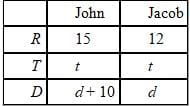John's travel during this "catch-up period" can be represented as 15t = d + 10
Jacob's travel during this "catch-up period" can be represented as 12t = d

If we solve these two simultaneous equations, we get:
15t = 12t + 10
3t = 10
t = 3 1/3 hours
Another way to approach this question is to note that when John begins to ride again, Jacob is 10 miles ahead. So John must make up those first 10 miles plus whatever additional distance Jacob has covered while both are riding. Since Jacob's additional distance at any given moment is 12t (measuring from the moment when John begins riding again) we can represent the distance that John has to make up as 12t + 10. We can also represent John's distance at any given moment as 15t. Therefore, 15t = 12t + 10, when John catches up to Jacob. We can solve this question as outlined above.

QUESTION: 8

Deb normally drives to work in 45 minutes at an average speed of 40 miles per hour. This week, however, she plans to bike to work along a route that decreases the total distance she usually travels when driving by 20% . If Deb averages between 12 and 16 miles per hour when biking, how many minutes earlier will she need to leave in the morning in order to ensure she arrives at work at the same time as when she drives?

Solution:

This standard rate problem will rely heavily on the formula RT=D, where R is the rate, T is the time and D is the distance traveled.
First, we should find the driving and biking distances:
If Deb drives for 45 minutes, or 0.75 hours, at a rate of 40mph, she drives a total distance of
(0.75)(40) = 30 miles.
If the bike route is 20% shorter than the driving route, the bike route is 30 – 30(0.2) = 30 – 6 = 24 miles.
Next, we need to determine how long it will take Deb to travel the route by bike.  She wants to ensure that she'll get to work by a particular time, so we want to calculate the longest possible time it could take her; therefore, we have to assume she will bike at the slowest end of the range of the speeds given: 12mph.  If she travels 24 miles at 12mph, it will take her 24/12 = 2 hours or 120 minutes.
If Deb normally takes 45 minutes to drive to work but could take up to 120 minutes to bike to work, then she must leave 120 – 45 = 75 minutes earlier than she normally does to ensure that she will arrive at work at the same time.

QUESTION: 9

Alex and Brenda both stand at point X. Alex begins to walk away from Brenda in a straight line at a rate of 4 miles per hour. One hour later, Brenda begins to ride a bicycle in a straight line in the opposite direction at a rate of R miles per hour. If R > 8, which of the following represents the amount of time, in terms of R, that Alex will have been walking when Brenda has covered twice as much distance as Alex?

Solution:

If we want Brenda's distance to be twice as great as Alex's distance, we can set up the following equation:

2(4T) = R(T – 1), where 4T is Alex's distance (rate × time) and R(T – 1) is Brenda's distance (since Brenda has been traveling for one hour less).

If we simplify this equation to isolate the T (which represents Alex's total time), we get:
2(4T) = R(T – 1)
8T = RT – R
R = RT – 8T
R = T(R – 8)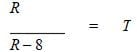This is choice C

QUESTION: 10

On Monday, Lou drives his ford escort with 28-inch tires, averaging x miles per hour. On Tuesday, Lou
switches the tires on his car to 32-inch tires yet drives to work at the same average speed as on Monday. What is the percent change from Monday to Tuesday in the average number of revolutions that Lou’s tires make per second?

Solution:

The key to solving this question lies in understanding the mathematical relationship that exists between the speed (s), the circumference of the tires (c) and the number of revolutions the tires make per second (r).  It makes sense that if you keep the speed of the car the same but increase the size of the tires, the number of revolutions that the new tires make per second should go down.  What, however, is the exact relationship?
Sometimes the best way to come up with a formula expressing the relationship between different variables is to analyze the labels (or units) that are associated with those variables.  Let’s use the following units for the variables in this question (even though they are slightly different in the question):
c: inches/revolution
s: inches/sec
r: revolutions/sec
The labels suggest:  (rev/sec) x (inches/rev) = (inches/sec), which means that rc = s.
When the speed is held constant, as it is in this question, the relationship rc = s becomes rc = k.  r and c are inversely proportional to one another.  When two variables are inversely proportional, it means that whatever factor you multiply one of the variables by, the other one changes by the inverse of that factor.  For example if you keep the speed constant and you double the circumference of the tires, the rev/sec will be halved.  In this way the product of c and r is kept constant.
In this question the circumferences of the tires are given in inches, the speed in miles per hour, and the rotational speed in revolutions per second.  However, the discrepancies here don’t affect the fundamental mathematical relationship of inverse proportionality: if the speed is kept constant, the rev/sec of the tires will change in an inverse manner to the circumference of the tires.
Let’s assign     c1 = initial circumference; c2 = new circumference
r1 = initial rev/sec ; r2 = new rev/sec
Since the speeds are held constant:
c1r1 = c2r2
r2 = (c1/c2)r1
r2 = (28/32)r1
r2 = (7/8)r1

If the new rev/sec is 7/8 of the previous rev/sec, this represents a 1/8 or 12.5% decrease and the correct answer is (B).  Relationships of inverse proportionality are important in any word problem on the GMAT involving a formula in the form of xy = z and in which z is held constant.Use Code STAYHOME200 and get INR 200 additional OFF Use Coupon Code